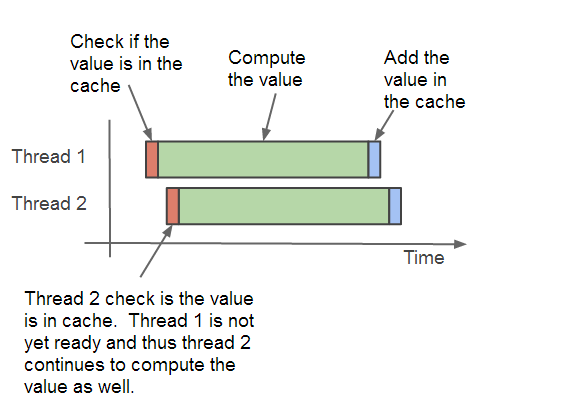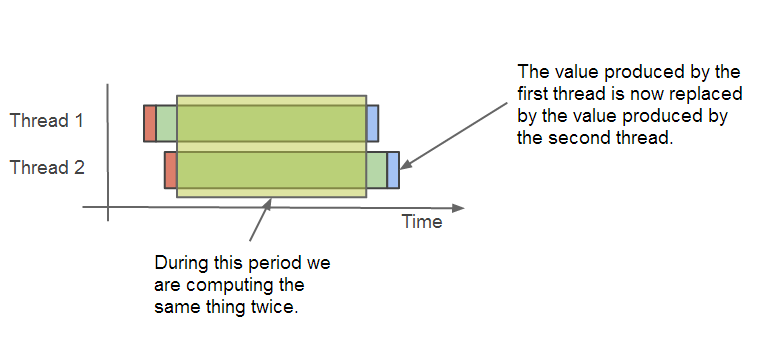# 缓存设计（1）

2017-03-17

## 简单实现

``````IF value in cached THEN
return value from cache
ELSE
compute value
save value in cache
return value
END IF
``````

``````import java.util.HashMap;
import java.util.Map;

public class NaiveCacheExample {

private Map<Long, Long> cache = new HashMap<>();

public NaiveCacheExample() {
// The base case for the Fibonacci Sequence
cache.put(0L, 1L);
cache.put(1L, 1L);
}

public Long getNumber(long index) {
// Check if value is in cache
if (cache.containsKey(index)) {
return cache.get(index);
}

// Compute value and save it in cache
long value = getNumber(index - 1) + getNumber(index - 2);
cache.put(index, value);
return value;
}
}
``````

1. 非线程安全

这个问题可以通过使用并发版本的 Map 来缓解，比如 ConcurrentMap。另外，也可以通过各种锁来限制 Map 的访问控制。各自权衡，不做评论

2. 同一个序列值可能被重复计算

假如两个线程需要获取一个相同的序列值，但是这个序列值并不在缓存里面， 此时，这两个线程都会各自去计算这个序列值，从而导致这个序列值被计算了两次。 如下图所示：这个缺陷不仅违背了缓存的初衷，同时也浪费了计算资源，降低了系统的吞吐量。如下图：缓存的目的是通过从缓存取值来减少计算需求，因此这个问题看起来无关紧要，但是却对系统资源（CPU，内存）有严重的影响。

3. 这种设计难以移植到其他场景应用中

这个缓存方案被硬编码为针对单一计算的实现，在此例中，是斐波那契生成器。 getNumber() 同时负责缓存数据和计算序列值，无法将这两种责任剥离开来。如果我们想将此缓存应用到别的场景，比如计算一个大整数是不是质数， 我们需要同时编写缓存逻辑和质数计算代码，结果导致我们不得不修改每一处已经使用或打算使用缓存的地方。

1. ConcurrentMap 的 putIfAbsent() 使用线程安全的方式将一个不在 map 内的新值添加到 map 内。
2. Future 的 get() 方法保证了同时只会有一个线程在计算某个序列值。
3. 最后，求值算法被抽象为 Callable，由于 Callable 可以任意实现，缓存方案就可以应用到任意场景。

## 线程安全的通用缓存方案

1. 缓存必须线程安全
2. 足够智能，当第一个线程还没有完成计算时，要避免第二个线程进行相同的计算。当第一个线程完成计算之后，第二个线程直接使用第一个线程计算得来的结果。
3. 将与斐波那契计算相关的代码全部挪出缓存，同时，使用泛型来适应不同的场景。

``````import java.util.concurrent.Callable;
import java.util.concurrent.ConcurrentHashMap;
import java.util.concurrent.ConcurrentMap;
import java.util.concurrent.ExecutionException;
import java.util.concurrent.Future;

public class GenericCacheExample<K, V> {

private final ConcurrentMap<K, Future<V>> cache = new ConcurrentHashMap<>();

private Future<V> createFutureIfAbsent(final K key, final Callable<V> callable) {
Future<V> future = cache.get(key);
if (future == null) {
future = cache.putIfAbsent(key, futureTask);
if (future == null) {
}
}
return future;
}

public V getValue(final K key, final Callable<V> callable) throws InterruptedException, ExecutionException {
try {
final Future<V> future = createFutureIfAbsent(key, callable);
return future.get();
} catch (final InterruptedException e) {
cache.remove(key);
throw e;
} catch (final ExecutionException e) {
cache.remove(key);
throw e;
} catch (final RuntimeException e) {
cache.remove(key);
throw e;
}
}

public void setValueIfAbsent(final K key, final V value) {
createFutureIfAbsent(key, new Callable<V>() {
@Override
public V call() throws Exception {
return value;
}
});
}

}
``````

`````` private Future<V> createFutureIfAbsent(final K key, final Callable<V> callable) {
//从 cache 中取得对应的 Future
Future<V> future = cache.get(key);
//如果 future 是 null，就新建一个 future，并添加草 cache 里面
if (future == null) {
future = cache.putIfAbsent(key, futureTask);
//putIfAbsent() 方法返回先前已经存在的 Future，
//如果返回的 future 是null，表明这个 key 在 cache 中没有对应的值。
//于是我们新建的 future 会被自动添加到 cache 里
if (future == null) {
//开始 futureTask，不然 future 无法开始执行
}
}
return future;
}
``````

``````  public V getValue(final K key, final Callable<V> callable) throws InterruptedException, ExecutionException {
try {
final Future<V> future = createFutureIfAbsent(key, callable);
return future.get();
} catch (final InterruptedException e) {
cache.remove(key);
throw e;
} catch (final ExecutionException e) {
cache.remove(key);
throw e;
} catch (final RuntimeException e) {
cache.remove(key);
throw e;
}
}
``````

Future#get() 方法可能会发生异常，这个时候我们应该将对应的 key 从缓存中移除，并将异常抛出给调用者来处理。

``````public void setValueIfAbsent(final K key, final V value) {
createFutureIfAbsent(key, new Callable<V>() {
@Override
public V call() throws Exception {
return value;
}
});
}
``````

## 实践：新的缓存方案

### 例子一，斐波那契序列求值

``````import java.util.concurrent.Callable;

import org.slf4j.Logger;
import org.slf4j.LoggerFactory;

public class FibonacciExample {

private static final Logger LOGGER = LoggerFactory.getLogger(FibonacciExample.class);

public static void main(final String[] args) throws Exception {
final long index = 12;
final FibonacciExample example = new FibonacciExample();
final long fn = example.getNumber(index);
FibonacciExample.LOGGER.debug("The {}th Fibonacci number is: {}", index, fn);
}

private final GenericCacheExample<Long, Long> cache = new GenericCacheExample<>();

public FibonacciExample() {
cache.setValueIfAbsent(0L, 1L);
cache.setValueIfAbsent(1L, 1L);
}

public long getNumber(final long index) throws Exception {
return cache.getValue(index, new Callable<Long>() {
@Override
public Long call() throws Exception {
FibonacciExample.LOGGER.debug("Computing the {} Fibonacci number", index);
return getNumber(index - 1) + getNumber(index - 2);
}
});
}
}
``````

``````Computing the 12 Fibonacci number
Computing the 11 Fibonacci number
Computing the 10 Fibonacci number
Computing the 9 Fibonacci number
Computing the 8 Fibonacci number
Computing the 7 Fibonacci number
Computing the 6 Fibonacci number
Computing the 5 Fibonacci number
Computing the 4 Fibonacci number
Computing the 3 Fibonacci number
Computing the 2 Fibonacci number
The 12th Fibonacci number is: 233
``````

### 例子二，虚拟的耗时任务

``````import java.util.concurrent.Callable;

import org.slf4j.Logger;
import org.slf4j.LoggerFactory;
import org.springframework.util.StopWatch;

public class FictitiousLongRunningTask {

private static final Logger LOGGER = LoggerFactory.getLogger(FictitiousLongRunningTask.class);

public static void main(final String[] args) throws Exception {

final StopWatch stopWatch = new StopWatch("Fictitious Long Running Task");
stopWatch.start("First Run");
stopWatch.stop();

stopWatch.start("Other Runs");
for (int i = 0; i < 100; i++) {
}
stopWatch.stop();

}

private final GenericCacheExample<String, Long> cache = new GenericCacheExample<>();

public long computeLongTask(final String key) throws Exception {
return cache.getValue(key, new Callable<Long>() {
@Override
public Long call() throws Exception {
FictitiousLongRunningTask.LOGGER.debug("Computing Fictitious Long Running Task: {}", key);
Thread.sleep(10000); // 10 seconds
return System.currentTimeMillis();
}
});
}
}
``````

``````Computing Fictitious Long Running Task: a
StopWatch 'Fictitious Long Running Task': running time (millis) = 10006; [First Run] took 10005 = 100%; [Other Runs] took 1 = 0%
``````